Home > CCA2 > Chapter 9 > Lesson 9.3.1 > Problem9-80

9-80.
1. Denae and Gina were trying to solve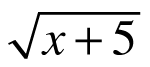+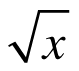= 5. They knew they would have to square the equation to get rid of the radicals, but when they tried it, they got x + 5 +2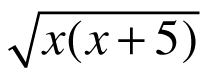+ x = 25, which did not look much easier to deal with. Gina had an idea.

2.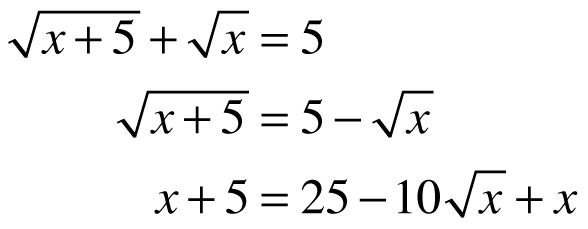Let’s start by putting the radicals on opposite sides of the equation and then squaring,” she said. Her work is shown at right.

3. But there’s still a radical!” she exclaimed, disappointed.

4. That’s okay,” said Denae, “there’s only one now, so we can get the term with the radical by itself and then square everything again.Homework Help ✎

1. Follow Denae’s advice and solve this equation.

2. Use Denae and Gina’s method to solve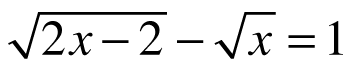.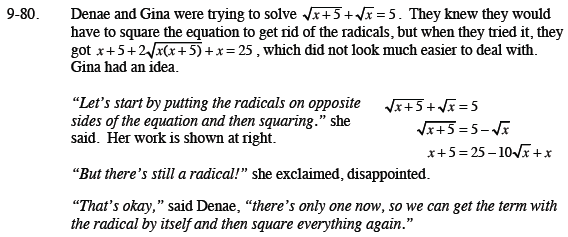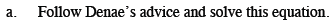$x+5=25-10\sqrt{x}+x$

$-20=-10\sqrt{x}$

$2=\sqrt{x}$

$?=x$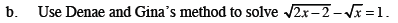$\sqrt{2x-2}=1+\sqrt{x}$

$\left(\sqrt{2x-2}\right)^{2}=\left(1+\sqrt{x}\right)^2$

$2x-2=1+1\sqrt{x}+1\sqrt{x}+x$

$x-3=2\sqrt{x}$

$\left(x-3\right)^2=\left(2\sqrt{x}\right)^2$

$x^2-3x-3x+9=4x$

Continue solving. Note that you will need to factor and use the Zero Product Property.
Be sure to check both of your answers in the original equation.